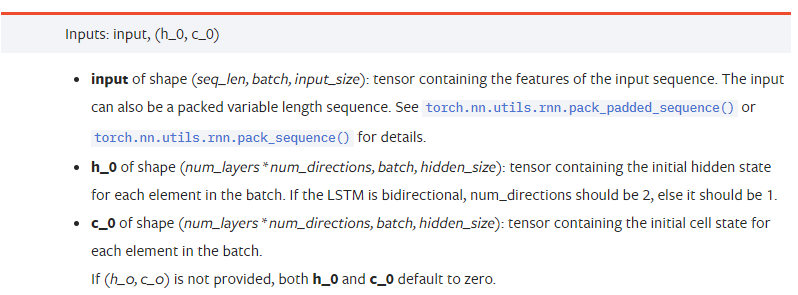# 神经网络与数字货币量化交易系列（1）——LSTM预测比特币价格

### 3.pytorch LSTM模型的参数

LSTM的参数：input_size: 输入向量x的特征大小,如果以收盘价预测收盘价,那么input_size=1;如果以高开低收预测收盘价,那么input_size=4
hidden_size: 隐含层大小
num_layers: RNN的层数
batch_first: 如果为True则输入维度的第一个为batch_size,这个参数也很让人困惑,下面将详细介绍。input: 具体输入的数据,是一个三维的tensor, 具体的形状为:(seq_len, batch, input_size)。其中,seq_len指序列的长度,即LSTM需要考虑多长时间的历史数据,注意这个指只是数据的格式,不是LSTM内部的结构,同一个LSTM模型可以输入不同seq_len的数据,都能给出预测的结果;batch指batch的大小,代表有多少组不同的数据;input_size就是前面的input_size。
h_0: 初始的hidden状态, 形状为(num_layers * num_directions, batch, hidden_size),如果时双向网络num_directions=2
c_0: 初始的cell状态, 形状同上, 可以不指定。output: 输出的形状 (seq_len, batch, num_directions * hidden_size),注意和模型参数batch_first有关
h_n: t = seq_len时刻的h状态,形状同h_0
c_n: t = seq_len时刻的c状态,形状同c_0

### 4.LSTM输入输出的简单例子

import pandas as pd
import numpy as np
import torch
import torch.nn as nn
import matplotlib.pyplot as plt


LSTM = nn.LSTM(input_size=5, hidden_size=10, num_layers=2, batch_first=True)

x = torch.randn(3,4,5)
# x的值为:
tensor([[[ 0.4657,  1.4398, -0.3479,  0.2685,  1.6903],
[ 1.0738,  0.6283, -1.3682, -0.1002, -1.7200],
[ 0.2836,  0.3013, -0.3373, -0.3271,  0.0375],
[-0.8852,  1.8098, -1.7099, -0.5992, -0.1143]],

[[ 0.6970,  0.6124, -0.1679,  0.8537, -0.1116],
[ 0.1997, -0.1041, -0.4871,  0.8724,  1.2750],
[ 1.9647, -0.3489,  0.7340,  1.3713,  0.3762],
[ 0.4603, -1.6203, -0.6294, -0.1459, -0.0317]],

[[-0.5309,  0.1540, -0.4613, -0.6425, -0.1957],
[-1.9796, -0.1186, -0.2930, -0.2619, -0.4039],
[-0.4453,  0.1987, -1.0775,  1.3212,  1.3577],
[-0.5488,  0.6669, -0.2151,  0.9337, -1.1805]]])

x的形状为(3,4,5),由于我们之前定义了batch_first=True, 此时的batch_size的大小为3, sqe_len为4, input_size为5。 x代表了第一个batch。

LSTM的输入输出的形状很容易让人迷惑,借助下图可以辅助理解:x = torch.randn(3,4,5)
h0 = torch.randn(2, 3, 10)
c0 = torch.randn(2, 3, 10)
output, (hn, cn) = LSTM(x, (h0, c0))
print(output.size()) #在这里思考一下,如果batch_first=False输出的大小会是多少?
print(hn.size())
print(cn.size())
#结果
torch.Size([3, 4, 10])
torch.Size([2, 3, 10])
torch.Size([2, 3, 10])

hn[-1] == output[-1] #第一个batch在hn最后一层的输出等于第一个batch在t=3时output的结果
hn[-1] == output[-1]
hn[-1] == output[-1]


### 5.准备比特币行情数据

import requests
import json

resp = requests.get('https://www.quantinfo.com/API/m/chart/history?symbol=BTC_USD_BITFINEX&resolution=60&from=1525622626&to=1562658565')
data = resp.json()
df = pd.DataFrame(data,columns = ['t','o','h','l','c','v'])
print(df.head(5))df.index = df['t'] # index设为时间戳
df = (df-df.mean())/df.std() # 数据的标准化,否则模型的Loss会非常大,不利于收敛
df['n'] = df['c'].shift(-1) # n为下一个周期的收盘价,是我们预测的目标
df = df.dropna()
df = df.astype(np.float32) # 改变下数据格式适应pytorch

seq_len = 10 # 输入10个周期的数据
train_size = 800 # 训练集batch_size
def create_dataset(data, seq_len):
dataX, dataY=[], []
for i in range(0,len(data)-seq_len, seq_len):
dataX.append(data[['o','h','l','c','v']][i:i+seq_len].values)
dataY.append(data['n'][i:i+seq_len].values)
return np.array(dataX), np.array(dataY)
data_X, data_Y = create_dataset(df, seq_len)
train_x = torch.from_numpy(data_X[:train_size].reshape(-1,seq_len,5)) #变化形状,-1代表的值会自动计算
train_y = torch.from_numpy(data_Y[:train_size].reshape(-1,seq_len,1))### 6.构造LSTM模型

class LSTM(nn.Module):
def __init__(self, input_size, hidden_size, output_size=1, num_layers=2):
super(LSTM, self).__init__()
self.rnn = nn.LSTM(input_size,hidden_size,num_layers,batch_first=True)
self.reg = nn.Linear(hidden_size,output_size) # 线性层,把LSTM的结果输出成一个值

def forward(self, x):
x, _ = self.rnn(x) # 如果不理解前向传播中数据维度的变化,可单独调试
x = self.reg(x)
return x

net = LSTM(5, 10) # input_size为5,代表了高开低收和交易量. 隐含层为10.

### 7.开始训练模型

criterion = nn.MSELoss() # 使用了简单的均方差损失函数
for epoch in range(600): # 由于速度很快,这里的epoch多一些
out = net(train_x) # 由于数据量很小, 直接拿全量数据计算
loss = criterion(out, train_y)
loss.backward() # 反向传播损失
optimizer.step() # 更新参数
print('Epoch: {:<3}, Loss:{:.6f}'.format(epoch+1, loss.item()))### 8.模型评价

p = net(torch.from_numpy(data_X))[:,-1,0] # 这里只取最后一个预测值作为比较
plt.figure(figsize=(12,8))
plt.plot(p.data.numpy(), label= 'predict')
plt.plot(data_Y[:,-1], label = 'real')
plt.legend()
plt.show()r = data_Y[:,-1][800:1000]
y = p.data.numpy()[800:1000]
r_change = np.array([1 if i > 0 else 0 for i in r[1:200] - r[:199]])
y_change = np.array([1 if i > 0 else 0 for i in y[1:200] - y[:199]])
print((r_change == y_change).sum()/float(len(r_change)))posted @ 2019-07-13 16:36  fmzcom  阅读(...)  评论(...编辑  收藏